#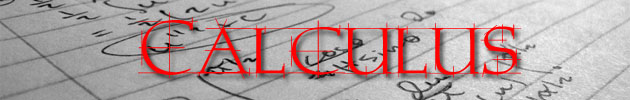Calculus is a central branch of mathematics. The word stems from the nascent development of mathematics: the early Greeks used pebbles arranged in patterns to learn arithmetic and geometry, and the Latin word for "pebble" is "calculus."

Calculus is built on two major complementary ideas, both of which rely critically on the concept of limits. The first is differential calculus, which is concerned with the instantaneous rate of change of quantities with respect to other quantities, or more precisely, the local behavior of functions. This can be illustrated by the slope of a function's graph. The second is integral calculus, which studies the accumulation of quantities, such as areas under a curve, linear distance traveled, or volume displaced. These two processes act inversely to each other, as shown by the fundamental theorem of calculus.

Examples of typical differential calculus problems include:

• finding the acceleration and velocity of a free-falling body at a particular moment
• finding the optimal number of units a company should produce to maximize its profit

Examples of integral calculus problems include:

• finding areas and volumes
• finding the amount of water pumped by a pump with a set power input but varying conditions of pumping losses and pressure
• finding the amount of parking lot plowed by a snowplow of given power with varying rates of snowfall

Today, calculus is used in every branch of the physical sciences, in computer science, in statistics, and in engineering; in economics, business, and medicine; and as a general method whenever the goal is an optimal solution to a problem that can be given in mathematical form.

For a much better overview and history of calculus, go to the

This site claims to be the web's most extensive mathematics resource, and a mathematician we know says it's a pretty good site. It doesn't deal with arithmetic, but the more advanced aspects of mathematics, like statistics and calculus.

Did you find this review helpful?
Parent Categories
Calculus & Statistics
27 Items found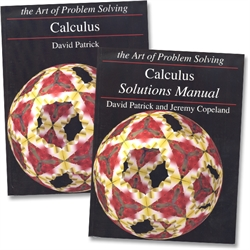Art of Problem Solving Calculus - Text & Solutions by David Patrick, Jeremy Copeland 2nd edition from Art of Problem Solving for 9th-12th grade in Art of Problem Solving (Location: MAT-AoPS) \$59.00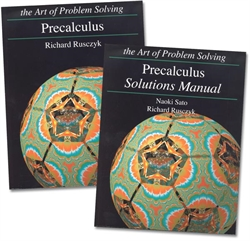Art of Problem Solving PreCalculus - Text & Solutions by Richard Rusczyk, Naoki Sato 2nd edition from Art of Problem Solving for 9th-12th grade in Art of Problem Solving (Location: MAT-AoPS) \$62.00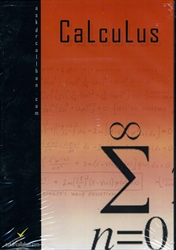Calculus - DVD from AskDrCallahan for 11th-12th grade in Calculus & Statistics (Location: MATR-CAL) \$145.00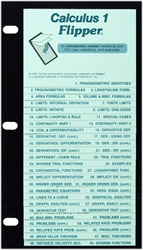Calculus 1 Flipper from Christopher Lee Publications for 11th-Adult in Math Flippers (Location: MATR-FLIP)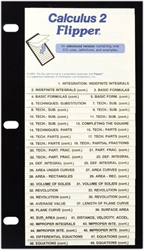Calculus 2 Flipper from Christopher Lee Publications for 12th-Adult in Math Flippers (Location: MATR-FLIP)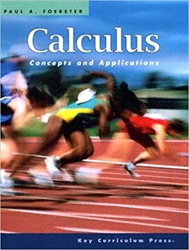Calculus: Concepts and Applications by Paul A. Foerster 2nd edition from Key Curriculum Press for 3rd grade in Calculus & Statistics (Location: MATR-CAL)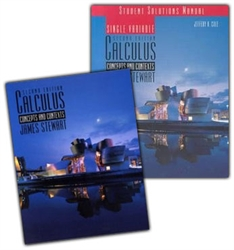Calculus: Concepts and Contexts 2nd edition from AskDrCallahan for 11th-12th grade in Calculus & Statistics (Location: MATR-CAL) \$30.00 (2 in stock)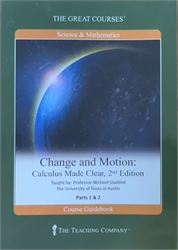Great Courses - Change and Motion by Professor Michael Starbird, teacher 2nd edition from The Teaching Company for 11th-Adult in Calculus & Statistics (Location: MATR-CAL) \$12.00 (1 in stock)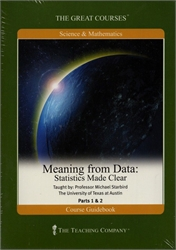Great Courses - Meaning from Data by Professor Michael Starbird, teacher 2nd edition from The Teaching Company for 11th-Adult in Calculus & Statistics (Location: MATR-CAL) \$12.00 (1 in stock)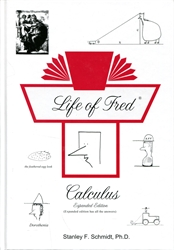Life of Fred: Calculus (Expanded Edition) Life of Fred by Stanley Schmidt 2nd edition from Polka Dot Publishing for 10th-12th grade in Life of Fred Math (Location: MAT-FRED) \$57.00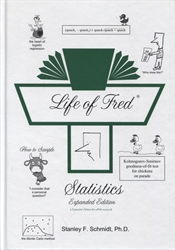Life of Fred: Statistics (Expanded Edition) by Stanley Schmidt Expanded from Polka Dot Publishing for 11th-12th grade in Life of Fred Math (Location: MAT-FRED) \$57.00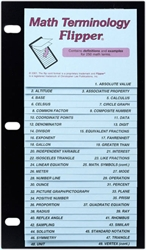Math Terminology Flipper from Christopher Lee Publications for 4th-10th grade in Math Flippers (Location: MATR-FLIP)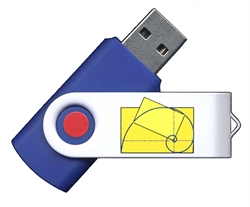Math Without Borders Calculus by David S. Chandler from Math Without Borders for 11th-12th grade in Math Without Borders (Location: MAT-MBB) \$129.00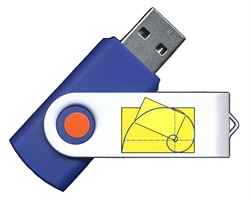Math Without Borders PreCalculus by David S. Chandler from Math Without Borders for 11th-12th grade in Math Without Borders (Location: MAT-MBB) \$129.00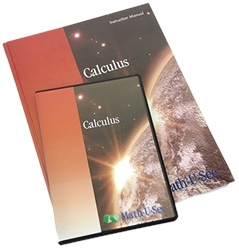Math-U-See Calculus - Instruction Pack from Math-U-See for 12th grade in Math-U-See (Location: MAT-MUS) \$103.00Math-U-See Calculus - Student Pack from Math-U-See for 12th grade in Math-U-See (Location: MAT-MUS) \$48.00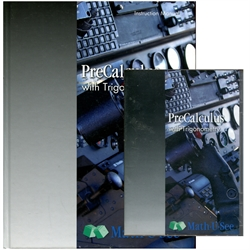Math-U-See PreCalculus - Instruction Pack from Math-U-See for 11th grade in Math-U-See (Location: MAT-MUS)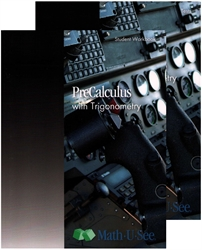Math-U-See PreCalculus - Student Pack from Math-U-See for 11th grade in Math-U-See (Location: MAT-MUS) \$48.00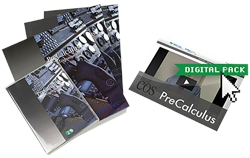Math-U-See PreCalculus Universal Set from Math-U-See for 11th grade in Math-U-See (Location: MAT-MUS) \$144.00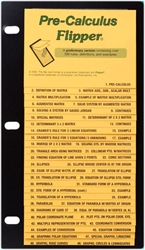Pre-Calculus Flipper from Christopher Lee Publications for 10th-Adult in Math Flippers (Location: MATR-FLIP)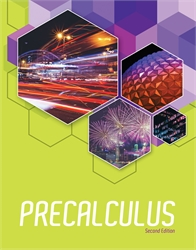Precalculus - Student Textbook 2nd edition from Bob Jones University Press for 12th grade in BJU Math (Location: MAT-BJU) \$68.89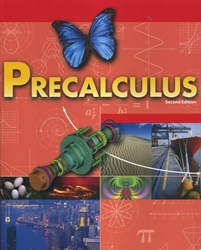Precalculus - Student Textbook (old) from Bob Jones University Press for 12th grade in BJU Math (Old Versions only) (Location: OBJU-MAT) \$25.00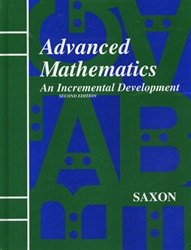Saxon Advanced Mathematics - Textbook by John Saxon 2nd edition from Saxon Publishers for 11th-12th grade in Saxon Math (Location: MAT-SAX)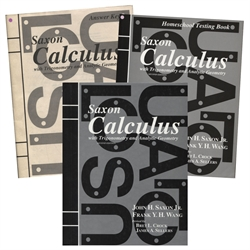Saxon Calculus - Home Study Kit 2nd edition from Saxon Publishers for 12th grade Cathy Duffy's 100 Top Picks in Saxon Math (Location: MAT-SAX) \$171.00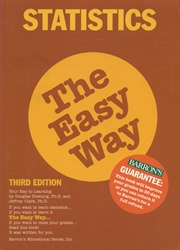Statistics - The Easy Way from Barron's for 11th-12th grade in Calculus & Statistics (Location: MATR-CAL) \$7.50 (1 in stock)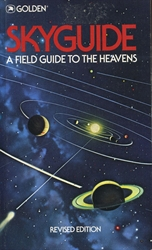Teaching Statistics: A Bag of Tricks by Andrew Gelman & Deborah Nolan from Oxford University for 11th-Adult in Calculus & Statistics (Location: MATR-CAL) \$10.00 (1 in stock)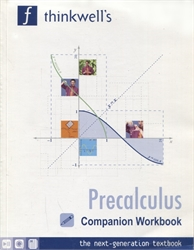Thinkwell's Precalculus Companion Workbook by Edward Burger from Thinkwell for 11th-12th grade in Calculus & Statistics (Location: MATR-CAL) \$39.00 (1 in stock)